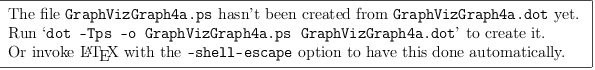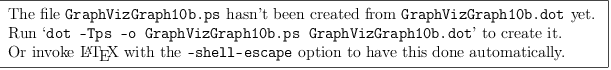login  home  contents  what's new  discussion  bug reports help  links  subscribe  changes  refresh  edit

## An Introduction to GraphViz

By Mihalis Tsoukalos on Wed, 2004-09-08 23:00.

How to use command-line tools and basic GraphViz utilities to produce graphs both simple and complex.

GraphViz is a collection of tools for manipulating graph structures and generating graph layouts. Graphs can be either directed or undirected. GraphViz offers both graphical and command-line tools. A Perl interface also is available, but it is not covered here for reasons of generality. Graphical tools are not going to be discussed in this article either. Instead, this article focuses on using GraphViz from the command line.

See more here: http://www.wickle.com/wikis/index.php/Graphviz_extension

The format of a GraphViz command is:

  \begin{latex}.
\digraph[Options]{Name}{Specification}
\end{latex}


Replace Options with something like scale=1.0 and Specifications with the actual graph, e.g. a->b; b->c. Replace Name with a name for your graph.

The LaTeX extension for GraphViz (see: LatexTemplate) produces files named Name.dot and Name.ps in the images working directory. Since this graph name is global, it is a good idea to prefix the name with the name of the page on which the graph occurs.

The follow graphs are generated by MathAction

My First Graph:

  \begin{latex}.
\psfrag{alpha}[cc][cc]{$\alpha$}
\psfrag{beta}[cc][cc]{$\beta$}
\psfrag{gamma}[cc][cc]{$\gamma$}
\digraph[scale=0.75]{GraphVizGraph1a}{rankdir=LR; alpha->beta; beta->gamma}
\end{latex}


Produces: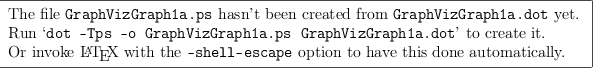A More Complex Example:

  \begin{latex}.
\digraph[scale=0.8]{GraphVizGraph2a}{
size ="4,4";
main [shape=box]; /* this is a comment */
main -> parse [weight=8];
parse -> execute;
main -> init [style=dotted];
main -> cleanup;
execute -> { make_string; printf}
init -> make_string;
edge [color=red];
main -> printf [style=bold,label="100 times"];
make_string [label="make a string"];
node [shape=box,style=filled,color=".7 .3 1.0"];
execute -> compare;
}
\end{latex}


Produces: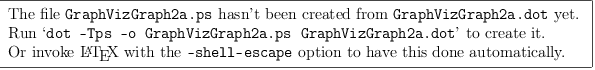## Here's One From

This:

  \begin{latex}.
\digraph{GraphVizGraph3a}{
node [shape = record];
node0 [ label ="<f0> | <f1> J | <f2> "];
node1 [ label ="<f0> | <f1> E | <f2> "];
node4 [ label ="<f0> | <f1> C | <f2> "];
node6 [ label ="<f0> | <f1> I | <f2> "];
node2 [ label ="<f0> | <f1> U | <f2> "];
node5 [ label ="<f0> | <f1> N | <f2> "];
node9 [ label ="<f0> | <f1> Y | <f2> "];
node8 [ label ="<f0> | <f1> W | <f2> "];
node10 [ label ="<f0> | <f1> Z | <f2> "];
node7 [ label ="<f0> | <f1> A | <f2> "];
node3 [ label ="<f0> | <f1> G | <f2> "];
"node0":f0 -> "node1":f1;
"node0":f2 -> "node2":f1;
"node1":f0 -> "node4":f1;
"node1":f2 -> "node6":f1;
"node4":f0 -> "node7":f1;
"node4":f2 -> "node3":f1;
"node2":f0 -> "node5":f1;
"node2":f2 -> "node9":f1;
"node9":f0 -> "node8":f1;
"node9":f2 -> "node10":f1;
}
\end{latex}


Gets you this: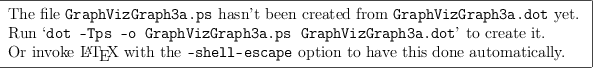Finally:

  \begin{latex}.
\digraph[scale=0.5]{GraphVizGraph4a}{
rankdir = LR;
node [shape=record, width=.1, height=.1];
node0 [label = "<p0> | <p1> | <p2> | <p3>
| <p4> | | ", height = 3];
node[ width=2 ];
node1 [label = "{<e> r0 | 123 | <p> }" ];
node2 [label = "{<e> r10 | 13 | <p> }" ];
node3 [label = "{<e> r11 | 23 | <p> }" ];
node4 [label = "{<e> r12 | 326 | <p> }" ];
node5 [label = "{<e> r13 | 1f3 | <p> }" ];
node6 [label = "{<e> r20 | 123 | <p> }" ];
node7 [label = "{<e> r40 | b23 | <p> }" ];
node8 [label = "{<e> r41 | 12f | <p> }" ];
node9 [label = "{<e> r42 | 1d3 | <p> }" ];
node0:p0 -> node1:e;
node0:p1 -> node2:e;
node2:p -> node3:e;
node3:p -> node4:e;
node4:p -> node5:e;
node0:p2 -> node6:e;
node0:p4 -> node7:e;
node7:p -> node8:e;
node8:p -> node9:e;
}
\end{latex}